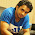# Short Trick of Calculating Square

I have already shared my technique to calculate squares here but today Gaurav Kaushik shared a trick to calculate squares.

How to calculate square?

Its too simple with some tricks. Lets move towards some examples:

Example 1: Find out the square of 12?
• Let divide 12 in two parts. Lets assume 1 is A while 2 is B.
• Now first find out the square of last digit. Here last digit is 2. So square of 2 is 4. Write down 4 in a order as i show u here__4.
• Now to find out the middle numbers of square we have a formula 2xAxB. Here A is 1 while B is 2. So according to formula 2x1x2=4. Write down this 4 in order_44.
• Now find out the square of first digit. Here first digit is 1. So square of 1 is 1. Now when we put this 1 in order then we find our answer 144.
Now we move to certain high digits.

Example 2: Find out square of 49?
• Let's divide 49 in two parts. Lets assume 4 is A while 9 is B.
• Now first find out the square of last digit. Here last digit is 9. So square of 9 is 81. But 81 is a two digit number so we use 1 only while use 8 as a carry forward for further calculation. So now we put 1 in an order as i show u here ___1.
• Now to find out the middle numbers of square we have a formula 2xAxB. Here A is 4 while B is 9.so according to formula 2x4x9= 72. As according to previous step we carry forward 8 so now we added 8 in 72 (8+72=80). Now again here we use 0 and carry forward 8 for next step. Write down 0 in order as i write down__01.
• Now find out the square of first digit. Here first digit is 4. So square of 4 is 16 as we all knows and in previous step we have a carry of 8 so after adding 8 in 16(8+16 =24) we get 24. Now write down 24 as i in order as i follows 2401.
Now we move to certain more higher level.

Example 3 : Find out square of 172?
• Let divide 172 in two parts. Let assume 17 is A while 2 is B.
• Now first find out the square of last digit. Here last digit is 2. So square of 2 is 4. Write down 4 in order as i shows u here____4.
• Now to find out the middle numbers of square we have a formula 2xAxB. Here A is 17 while B is 2 so according to formula 2x17x2=68. 68 is a two digit number so we use 8 only while use 6 as a carry forward for further calculation. So now we put 8 in order as i shows u here___84.
• Now we find out the square of A . As we knows A is 17 so square of 17 is 289. And as we knows according to previous step 6 is carry forwarded so we added 6 in 289 (6+289=295) so we get the digit 295. Now we put 295 in order as i shows u here 29584.
Just try out all steps by practice. It will not consume much time.#### What's trending in BankExamsToday

Smart Prep Kit for Banking Exams by Ramandeep Singh - Download here

##### Join 40,000+ readers and get free notes in your email#### 13 comments:

1.1.kindly correct the title of this post. square instead of square root

2.Thak u sir waiting for another post like this

3.Thank You Sir

4.Remember...
(a+b)^2 = a^2+2ab+b^2

& Very Good Trick...

5.6.Really superb keep it up tricks sharing

7.Excellent!!

8.Thanku sir,very helpful

9.really nice

10.This is traditional formula usage (a+b)^2. Not a trick. Don't pervert by false title's

11.Ibps clerk 3 result will declare on tomorrow
Source ibps

12.Gud trick...

Thanks for commenting. Follow us on Telegram. Search BankExamsToday